# Day 05 python进阶

10 篇文章 0 订阅

Python进阶鸭

PEP8代码规范
- import:

- 代码编排:

- 注释编排：

- 命名风格：

**Python中 * 和 的区别

• 函数定义
def foo(*args,**kwargs):
pass


Python中，（ *）会把接收到的参数形成一个元组，而（ ** ）则会把接收到的参数存入一个字典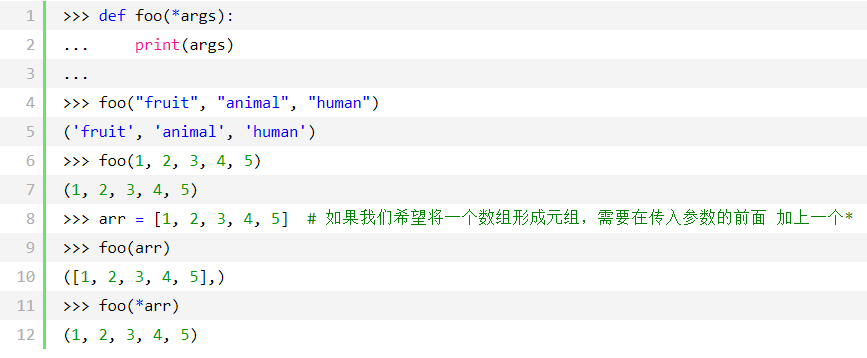（**）将接收到的参数存入一个字典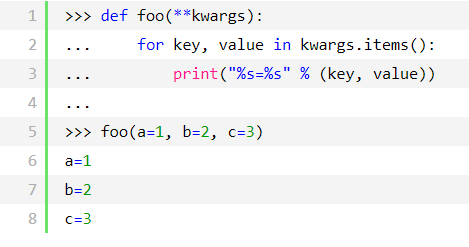（ * ）和（ ** ）一起使用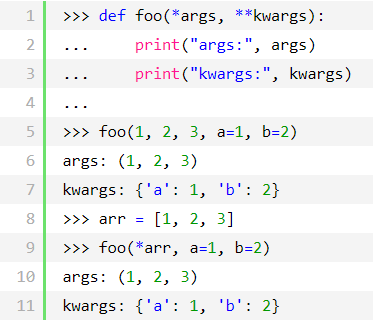Python的赋值和引用
== ，is : ==判断的是值，is判断的是内存地址（即对象的id）
Python的迭代器与生成器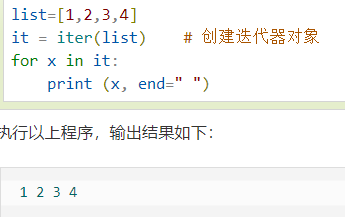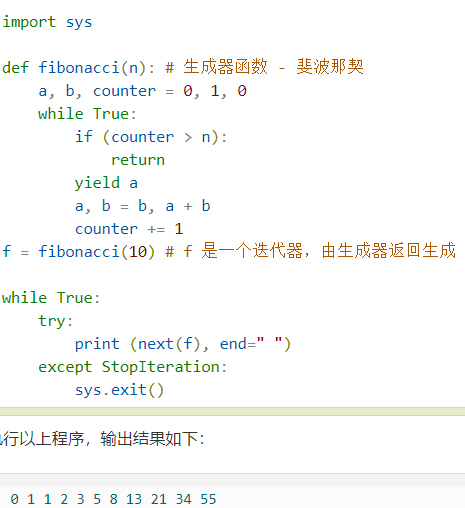python装饰器（fuctional decorators）就是用于拓展原来函数功能的一种函数，目的是在不改变原函数名(或类名)的情况下，给函数增加新的功能。

import time
def f():
print("hello")
time.sleep(1)
print("world")


import time
def f():
start_time = time.time()
print("hello")
time.sleep(1)
print("world")
end_time = time.time()

execution_time = (end_time - start_time)*1000
print("time is %d ms" %execution_time)


import time

def deco(func):
start_time = time.time()
f()
end_time = time.time()
execution_time = (end_time - start_time)*1000
print("time is %d ms" %execution_time)

def f():
print("hello")
time.sleep(1)
print("world")

if __name__ == '__main__':

deco(f)
print("f.__name__ is",f.__name__)
print()


import time

def deco(f):
def wrapper():
start_time = time.time()
f()
end_time = time.time()
execution_time = (end_time - start_time)*1000
print("time is %d ms" %execution_time )
return wrapper

@deco
def f():
print("hello")
time.sleep(1)
print("world")

if __name__ == '__main__':
f()


f()函数就相当于被注入了计时功能，现在只要调用f()，它就已经变身为“新的功能更多”的函数了,

（不需要重复执行原函数）。

import time

def deco(f):
def wrapper(a,b):
start_time = time.time()
f(a,b)
end_time = time.time()
execution_time = (end_time - start_time)*1000
print("time is %d ms" % execution_time)
return wrapper

@deco
def f(a,b):
print("be on")
time.sleep(1)
print("result is %d" %(a+b))

if __name__ == '__main__':
f(3,4)


import time

def deco(f):
def wrapper(*args, **kwargs):
start_time = time.time()
f(*args, **kwargs)
end_time = time.time()
execution_time_ = (end_time - start_time)*1000
print("time is %d ms" %execution_time)
return wrapper

@deco
def f(a,b):
print("be on")
time.sleep(1)
print("result is %d" %(a+b))

@deco
def f2(a,b,c):
print("be on")
time.sleep(1)
print("result is %d" %(a+b+c))

if __name__ == '__main__':
f2(3,4,5)
f(3,4)


import time

def deco01(f):
def wrapper(*args, **kwargs):
print("this is deco01")
start_time = time.time()
f(*args, **kwargs)
end_time = time.time()
execution_time = (end_time - start_time)*1000
print("time is %d ms" % execution_time)
print("deco01 end here")
return wrapper

def deco02(f):
def wrapper(*args, **kwargs):
print("this is deco02")
f(*args, **kwargs)

print("deco02 end here")
return wrapper

@deco01
@deco02
def f(a,b):
print("be on")
time.sleep(1)
print("result is %d" %(a+b))

if __name__ == '__main__':
f(3,4)



this is deco01
this is deco02
hello，here is a func for add :
result is 7
deco02 end here
time is 1003 ms
deco01 end here


07-3112308-2027
07-16530
03-31292
09-18132
07-21106
03-07169
04-2527
08-2048
04-29206
08-0647
08-0575
08-1481
08-01187
09-0547
07-2742
07-29111
08-1160点击重新获取扫码支付余额充值